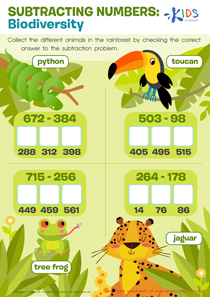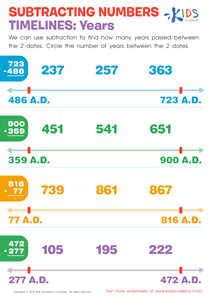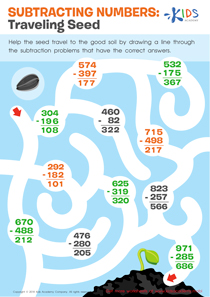# Math Lesson - Subtract within 1,000 Understanding what it means to borrow from 2 places, Grade 2

• ### Activity 1 / Subtracting Numbers: Biodiversity WorksheetSubtraction can only be made enjoyable and easier to understand for your students if you regularly engage them in simple tasks, and use worksheets such as this one with colorful print and enjoyable concepts. First, look at the animals in this worksheet with your students. Ask them if they can identify the animals, and then read the names of each animal carefully to them. Now, help them collect the different animals in the rainforest by checking the correct answer to the subtraction problem.

• ### Activity 2 / Subtracting Numbers Timelines: Years WorksheetYears are very important in keeping track of different historical events, and also in knowing how much time has passed. In this exercise, there are four mathematical problems which your child needs to solve in order to arrive at a conclusive answer. With this worksheet, we can use subtraction to find how many years passed between the 2 dates. Help your child circle the number of years between the 2 dates.

• ### Activity 3 / Subtracting Numbers: Traveling Seeds WorksheetIf you have introduced your students to subtracting equations, then you will need this simple mathematics worksheet to help them practice some of what they have learnt, and get better with subtracting figures. This printout uses compelling images to engage your student’s mind. Help the seed in the picture travel to the good soil by drawing a line through the subtraction problems with the correct answers. Solve some math with your students, and help them complete this simple maze.

?